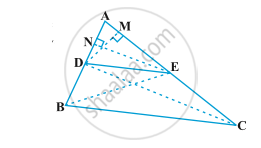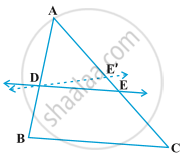Similarity of Triangles

definition

What can you say about the similarity of two triangles?
Two triangles are similiar, if
(i) their corresponding angles are equal and
(ii) their corresponding sides are in the same ratio (or proportion).

notes

Note that if corresponding angles of two triangles are equal, then they are known as equiangular triangles.
A famous Greek mathematician Thales gave an important truth relating to two equiangular triangles which is as follows: The ratio of any two corresponding sides in two equiangular triangles is always the same.
It is believed that he had used a result called the Basic Proportionality Theorem (now known as the Thales Theorem) for the same.

theorem

1)Thales Theorem or Basic Proportionality Theorem(BPT): If a line is drawn parallel to one side of a triangle to intersect the other two sides in distinct points, the other two sides are divided in the same ratio.Given: DE || BC
Construction: Draw EN ⊥ AB and DM ⊥ AC. Also join B to E and D to C.
To Prove: "AD"/"DB" = "AE"/"EC"
Proof: Now we know that area of triangle= (1/2 × base × height)
area of ∆ ADE = 1/2× "AD"× "EN"

So, ar(ADE)= 1/2 × "AD" × "EN"

Similarly, ar(BDE)= 1/2 × "DB" × "EN"

Here in ∆ BDE, the height is EN, because if we extend the base DB to BA then we get EN ⊥ BA.
If we take ratios of areas of triangles,

"ar(ADE)"/ "ar(BDE)"= (1/2 × "AD" × "EN")/ (1/2 × "DB" × "EN")

Therefore, "ar(ADE)"/ "ar(BDE)"= "AD"/"DB" .....eq1

Now, "ar(ADE)" = 1/2 × "AE" × "DM"

and, "ar(DCE)"= 1/2 × "EC" × "DM"

Here again, in ∆DCE, the height is DM, because if we extend the base EC to CA then we get DM ⊥ CA.
If we take ratios of areas of triangles,

"ar(ADE)"/"ar(DCE)"= "1/2 × AE × DM"/ "1/2 × EC × DM"

"ar(ADE)"/"ar(DCE)"= "AE"/"EC" ........eq2

We already know that, if two triangles re on the same base and between the same parallels then the areas of that two triangles are same.
Therefore, ar(BDE)=ar(DCE) ......eq3
From eq2 and eq3

"ar(ADE)"/"ar(BDE)"= "AE"/"EC" .......eq4

From eq1 and eq4

"AD"/"DB" = "AE"/"EC"

Hence proved.

2) Converse of Thales Theorem: If a line divides any two sides of a triangle in the same ratio, then the line is parallel to the third side.Given: "AD"/"DB" = "AE"/"EC"

Construction: Draw DE′ || BC
To prove: DE || BC
Proof: Let DE′ || BC  ......(By construction)

"AD"/"DB" = "AE′"/"E′C" ........eq1 (By BPT)

But, "AD"/"DB" = "AE"/"EC" ..........eq2 (Given)

From eq1 and eq2

"AE′"/"E′C" = "AE"/"EC"

"AE′"/"E′C" +1 = "AE"/"EC" +1

"AE′+E′C"/ "E′C" = "AE+EC"/ "EC"

"AC"/"E′C"= "AC"/"EC"

"EC" = "E′C"

This is possible only when E′ and E coincide
Therefore, DE || BC
Hence proved.

If you would like to contribute notes or other learning material, please submit them using the button below.

Shaalaa.com

Triangles part 3 (Thales theorem Activity) [00:04:14]
S
0%